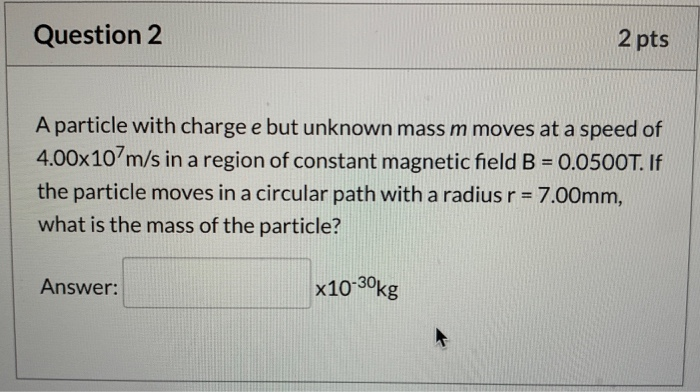# Question 2 2 pts A particle with charge e but unknown mass m moves at a...

###### Question:Question 2 2 pts A particle with charge e but unknown mass m moves at a speed of 4.00x107m/s in a region of constant magnetic field B = 0.0500T. If the particle moves in a circular path with a radius r = 7.00mm, what is the mass of the particle? Answer: x10-30kg

#### Similar Solved Questions

##### Let A = {a, b, c, d, e}, B = {a, b, e}, C = {d,...
Let A = {a, b, c, d, e}, B = {a, b, e}, C = {d, e, f}, and U= {a, b, c, d, e, f}. List the elements of: a. B times C b. C times C^c...
##### How do you evaluate sin ((5pi)/4)?
How do you evaluate sin ((5pi)/4)?...